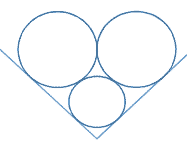# Mickey's Super-ears

Geometry Level 5Two lines intersect each other forming a right angle. A unit circle (radius 1) is tangent to both of these lines. Two equal size circles with radius $R>1$ are:
(1) tangent to this circle,
(2) tangent to each other, and
(3) each tangent to one of the lines,

as shown in the figure above.

Calculate $R$ to two decimal places.

×

Problem Loading...

Note Loading...

Set Loading...